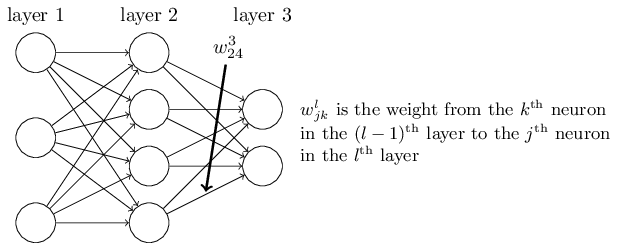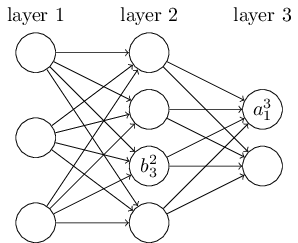## Explaination and Math

1,3 2,4Important: If my notes aren’t visible, please go the the individual page link at imgur:

## Notation used

Weights Biases (star)### Formulas to be further used in code :### Code

%matplotlib inline
import numpy as np
import random
import matplotlib.pyplot as plt
import json
import sys

# helper functions (here, activation functions)
def sigmoid(z):
"""the sigmoid activation function"""
return 1/(1+np.exp(-1*x))

def sigmoid_prime(x):
"""the derivative of the sigmoid function"""
return sigmoid(z)*(1-sigmoid(z))

def relu(z):
"""the ReLU activation function"""
return np.maximum(0,z)

def one_hot_encoding(j):
"""One hot encode to a 10-dimensional unit vector with prediction"""
encoded_vec = np.zeroes((10, 1))
encoded_vec[j] = 1.0
return ohe

# loss functions : here I'll try using both CrossEntropyLoss (losLoss) and
# QuadraticLoss functions to compare their performance.
# employed decent OOP practices

class CrossEntropyCost(object):

@staticmethod
def func(a, y):
"""
return : cost associated with input a and desired output y
sometime, when a = y, the formula for CrossEntropy returns NaN
Formula : (1-y)*np.log(1-a)
hence, np.nan_to_num is used to convert NaN's to (0.0)
"""
return np.sum(np.nan_to_numn(-y * np.log(a) - (1-y) * np.log(1-a)))

@staticmethod
def delta(a, y, z):
return (a - y)

class MSE_cost(object):

@staticmethod
def func(a, y):
"""return : cost associated with input a and desired output y"""
return np.linalg.norm(a - y) * 0.5 ** 2

@staticmethod
def delta(a, y, z):
z is the value of the neuron. from our derivation
"""
return (a - y) * sigmoid_prime(z)

class NN(object):

def __init__(self, size, cost = CrossEntropyCost):
"""
list::size : number of neurons in respective layers of the network
weights and biases are generated randomly through Gaussian Distribution with zero mean and variance of 1.
"""
self.n_layers = len(size)
self.size = size
# initializing weights only for 2nd to last layer since 1st layer is input layer (lacks weights)
self.biases = [np.random.randn(y, 1) for y in self.size[1:]]
self.weights = [np.random.randn(y, x)/np.sqrt(x)
for x, y in zip(self.size[:-1], self.size[1:])]
self.cost = cost

def forward_propagation(self, a):
"""The neuron calculation formula : Wa+b"""
for w, b in zip(self.weights, self.biases):
a = sigmoid(np.dot(w, a) + b)
return a

def back_propagation(self, x, y):
"""
return : (del_w, del_b) , the gradient for the cost function
del_w and del_b are layer-by-layer lists of numpy arrays.
Warning : negative indices would be heavily utilized
"""
del_w = [np.zeros(w.shape) for w in self.weights]
del_b = [np.zeroes(b.shape) for b in self.biases]
# forward prop
curr_activation = x
activations = [x] # store all activations by layer, remember chain-rule
z_lis = [] # store all z values for layers, remember tree structure from notes
for w, b in zip(self.weights, self.biases):
z = np.dot(w, curr_activation) + b
curr_activation = sigmoid(z)
z_lis.append(z)
activations.append(curr_activation)
# backward pass : calculating cost by taking last elems of a, z lists
delta = (self.cost).delta(activations[-1], y, z_lis[-1])
del_w[-1] = np.dot(delta, activations[-2].transpose())
del_b[-1] = delta

# going back all layers
for l in range(2, self.n_layers):
z = z_lis[-l]
del_sigmoid = sigmoid_prime(z)
delta = np.dot(self.weights[-l+1].transpose(), delta) * del_sigmoid
del_w[-l] = np.dot(delta, activations[-l-1].transpose())
del_b[-l] = delta
return (del_w, del_b)

def initialize_weight(self):
"""
initialize weights using Gaussian Distribution with mean 0 and SD  1
over sqrt of number of weights connecting same neuron
initialize biases using Gaussian Distribution with mean 0 and SD 1
"""
self.weights = [np.random.randn(y, x)/np.sqrt(x) for x, y in
zip(self.size[:-1], self.size[:-1])]
self.biases = [np.random.randn(y, 1) for y in self.size[1:]]

def trainer(self, training_data, epochs, m_bs, eta, lmbda, eval_data=None,
print_eval_cost=False, print_eval_acc=False, print_train_cost=False,
print_train_acc=False):
"""
Train the neural network using mini-batch stochastic gradient
descent.
"""
if eval_data:
n_data = sum(1 for _ in eval_data)
n = sum(1 for _ in training_data)
eval_cost, eval_acc = [], []
train_cost, train_acc = [], []
for c in range(epochs):
random.shuffle(training_data)
mini_batches = [training_data[k:k+m_bs] for k in
range(0, n, m_bs)]
for mini_batch in mini_batches:
self.update_mini_batch(mini_batch, eta, lmbda, len(training_data))
print('Training : Epoch % complete.' % c)

if print_train_cost:
acc = self.accuracy(training_data, convert=True)
train_acc.append(acc)
print('Accuracy on training data : {} / {}'.format(acc, n))
if print_train_cost:
cost = self.total_cost(training_data, lmbda)
train_cost.append(cost)
print('Cost on training data : {}'.format(cost))
if print_eval_acc:
acc = self.accuracy(eval_data)
eval_acc.append(acc)
print('Accuracy on training data : {} / {}'.format(acc, n))
if print_eval_cost:
cost = self.total_cost(eval_data, lmbda, convert=True)
train_cost.append(cost)
print('Cost on training data : {}'.format(cost))
print()
return (eval_cost, eval_acc, train_cost, train_acc)

def update_mini_batch(self, mini_batch, eta, lmbda, n):
"""Update the network's weights and biases by applying gradient
descent using backpropagation to a single mini batch.mini_batch is a list of tuples (x, y), eta is the
learning rate, lmbda is the regularization parameter, and
n is the total size of the training data set.
"""
nabla_b = [np.zeros(b.shape) for b in self.biases]
nabla_w = [np.zeros(w.shape) for w in self.weights]
for x, y in mini_batch:
delta_nabla_b, delta_nabla_w = self.backprop(x, y)
nabla_b = [nb+dnb for nb, dnb in zip(nabla_b, delta_nabla_b)]
nabla_w = [nw+dnw for nw, dnw in zip(nabla_w, delta_nabla_w)]
self.weights = [(1-eta*(lmbda/n))*w-(eta/len(mini_batch))*nw
for w, nw in zip(self.weights, nabla_w)]
self.biases = [b-(eta/len(mini_batch))*nb
for b, nb in zip(self.biases, nabla_b)]

def accuracy(self, data, convert=False):
"""Return the number of inputs in data for which the neural
network outputs the correct result. The neural network's
output is assumed to be the index of whichever neuron in the
final layer has the highest activation.
The flag convert should be set to False if the data set is
validation or test data (the usual case), and to True if the
data set is the training data.
"""
if convert:
results = [(np.argmax(self.feedforward(x)), np.argmax(y))
for (x, y) in data]
else:
results = [(np.argmax(self.feedforward(x)), y)
for (x, y) in data]
return sum(int(x == y) for (x, y) in results)

def total_cost(self, data, lmbda, convert=False):
"""Return the total cost for the data set data.
"""
cost = 0.0
for x, y in data:
a = self.feedforward(x)
if convert: y = vectorized_result(y)
cost += self.cost.fn(a, y)/len(data)
cost += 0.5*(lmbda/len(data))*sum(
np.linalg.norm(w)**2 for w in self.weights)
return cost

def save(self, filename):
"""Save the neural network to the file filename."""
data = {"size": self.size,
"weights": [w.tolist() for w in self.weights],
"biases": [b.tolist() for b in self.biases],
"cost": str(self.cost.__name__)}
f = open(filename, "w")
json.dump(data, f)
f.close()


# this python code to load MNIST Data is by Michael Nielsen.
import _pickle as cPickle
import gzip

# Third-party libraries
import numpy as np

"""Return the MNIST data as a tuple containing the training data,
the validation data, and the test data.
The training_data is returned as a tuple with two entries.
The first entry contains the actual training images.  This is a
numpy ndarray with 50,000 entries.  Each entry is, in turn, a
numpy ndarray with 784 values, representing the 28 * 28 = 784
pixels in a single MNIST image.
The second entry in the training_data tuple is a numpy ndarray
containing 50,000 entries.  Those entries are just the digit
values (0...9) for the corresponding images contained in the first
entry of the tuple.
The validation_data and test_data are similar, except
each contains only 10,000 images.
This is a nice data format, but for use in neural networks it's
helpful to modify the format of the training_data a little.
That's done in the wrapper function load_data_wrapper(), see
below.
"""
f = gzip.open('mnist.pkl.gz', 'rb')
training_data, validation_data, test_data = cPickle.load(f, encoding='latin1')
f.close()
return (training_data, validation_data, test_data)

"""Return a tuple containing (training_data, validation_data,
test_data). Based on load_data, but the format is more
convenient for use in our implementation of neural networks.
In particular, training_data is a list containing 50,000
2-tuples (x, y).  x is a 784-dimensional numpy.ndarray
containing the input image.  y is a 10-dimensional
numpy.ndarray representing the unit vector corresponding to the
correct digit for x.
validation_data and test_data are lists containing 10,000
2-tuples (x, y).  In each case, x is a 784-dimensional
numpy.ndarry containing the input image, and y is the
corresponding classification, i.e., the digit values (integers)
corresponding to x.
Obviously, this means we're using slightly different formats for
the training data and the validation / test data.  These formats
turn out to be the most convenient for use in our neural network
code."""
training_inputs = [np.reshape(x, (784, 1)) for x in tr_d]
training_results = [vectorized_result(y) for y in tr_d]
training_data = zip(training_inputs, training_results)
validation_inputs = [np.reshape(x, (784, 1)) for x in va_d]
validation_data = zip(validation_inputs, va_d)
test_inputs = [np.reshape(x, (784, 1)) for x in te_d]
test_data = zip(test_inputs, te_d)
return (training_data, validation_data, test_data)

def vectorized_result(j):
"""Return a 10-dimensional unit vector with a 1.0 in the jth
position and zeroes elsewhere.  This is used to convert a digit
(0...9) into a corresponding desired output from the neural
network."""
e = np.zeros((10, 1))
e[j] = 1.0
return e

training_data, validation_data, test_data = load_data_wrapper()

learner = NN([784, 30, 10])

learner.trainer(training_data, 30, 10, 3.0, 0.1, eval_data=test_data)

---------------------------------------------------------------------------
TypeError                                 Traceback (most recent call last)
<ipython-input-44-742e24694131> in <module>
----> 1 learner.trainer(training_data, 30, 10, 3.0, 0.1, eval_data=test_data)

<ipython-input-40-6f3c6ca52295> in trainer(self, training_data, epochs, m_bs, eta, lmbda, eval_data, print_eval_cost, print_eval_acc, print_train_cost, print_train_acc)
79         train_cost, train_acc = [], []
80         for c in range(epochs):
---> 81             random.shuffle(training_data)
82             mini_batches = [training_data[k:k+m_bs] for k in
83                            range(0, n, m_bs)]

~/anaconda3/envs/fastai/lib/python3.7/random.py in shuffle(self, x, random)
273         if random is None:
274             randbelow = self._randbelow
--> 275             for i in reversed(range(1, len(x))):
276                 # pick an element in x[:i+1] with which to exchange x[i]
277                 j = randbelow(i+1)

TypeError: object of type 'zip' has no len()

## Other Resources to dive more into Backpropagation Calculus

With this, I end this post. It was really intense, trying to comprehend the math behind Backpropagation in a single layer and then expanding the knowledge over to deep learning neural networks. 3B1B's video on Backpropagation was really intuitive to help me visualise the working in order to write this code.